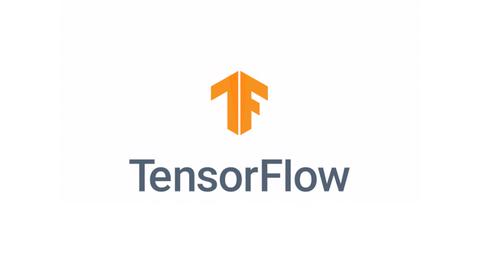TensorFlow2.0教程-自定义训练实战(非tf.keras)

Doit：最全Tensorflow2.0 入门教程持续更新zhuanlan.zhihu.com导入相关库

from __future__ import absolute_import, division, print_function, unicode_literals
import os
import matplotlib.pyplot as plt
!pip install -q tensorflow==2.0.0-alpha0
import tensorflow as tf
print('tf version:', tf.__version__)
print('eager execution:', tf.executing_eagerly())
tf version: 2.0.0-alpha0
eager execution: True

鸢尾花分类问题

train_dataset_fp = tf.keras.utils.get_file(fname=os.path.basename(train_dataset_url),
origin=train_dataset_url)
print('下载数据至：', train_dataset_fp)

检查数据

120,4,setosa,versicolor,virginica
6.4,2.8,5.6,2.2,2
5.0,2.3,3.3,1.0,1
4.9,2.5,4.5,1.7,2
4.9,3.1,1.5,0.1,0

column_names = ['sepal_length', 'sepal_width', 'petal_length', 'petal_width', 'species']
# 获取特征和标签名
feature_name = column_names[:-1]
label_name = column_names[-1]

• 0：Iris setosa:
• 1：Iris versicolor
• 2：Iris virginica
class_names = ['Iris setosa', 'Iris versicolor', 'Iris virginica']

创建一个 tf.data.Dataset

TensorFlow的数据集API处理许多将数据加载到模型中的常见情况。这是一个高级API，用于读取数据并将其转换为用于训练的数据类型。

batch_size=32
train_dataset = tf.data.experimental.make_csv_dataset(
train_dataset_fp,
batch_size,
column_names=column_names,
label_name=label_name,
num_epochs=1
)

features, labels = next(iter(train_dataset))
print(features)
OrderedDict([('sepal_length', <tf.Tensor: id=64, shape=(32,), dtype=float32, numpy=
array([7.6, 6.9, 7.2, 5. , 6.7, 4.8, 5.4, 5.1, 7.7, 6. , 6.3, 7.4, 5.2,
7.2, 6.7, 6.1, 5. , 4.9, 6.2, 4.5, 6.6, 6. , 5.5, 6.3, 4.8, 6.7,
6.1, 5.6, 7.3, 6.9, 5.7, 6.3], dtype=float32)>), ('sepal_width', <tf.Tensor: id=65, shape=(32,), dtype=float32, numpy=
array([3. , 3.2, 3.6, 2.3, 3. , 3. , 3.9, 3.7, 3. , 2.2, 2.3, 2.8, 2.7,
3.2, 3.1, 2.8, 3.4, 3.1, 2.8, 2.3, 3. , 3. , 3.5, 3.3, 3.4, 3. ,
2.8, 2.9, 2.9, 3.1, 3.8, 2.5], dtype=float32)>), ('petal_length', <tf.Tensor: id=62, shape=(32,), dtype=float32, numpy=
array([6.6, 5.7, 6.1, 3.3, 5.2, 1.4, 1.3, 1.5, 6.1, 5. , 4.4, 6.1, 3.9,
6. , 5.6, 4. , 1.6, 1.5, 4.8, 1.3, 4.4, 4.8, 1.3, 6. , 1.6, 5. ,
4.7, 3.6, 6.3, 4.9, 1.7, 5. ], dtype=float32)>), ('petal_width', <tf.Tensor: id=63, shape=(32,), dtype=float32, numpy=
array([2.1, 2.3, 2.5, 1. , 2.3, 0.3, 0.4, 0.4, 2.3, 1.5, 1.3, 1.9, 1.4,
1.8, 2.4, 1.3, 0.4, 0.1, 1.8, 0.3, 1.4, 1.8, 0.2, 2.5, 0.2, 1.7,
1.2, 1.3, 1.8, 1.5, 0.3, 1.9], dtype=float32)>)])

plt.scatter(features['petal_length'],
features['sepal_length'],
c=labels,
cmap='viridis')

plt.xlabel("Petal length")
plt.ylabel("Sepal length")
plt.show()

def pack_features_vector(features, labels):
features = tf.stack(list(features.values()), axis=1)
return features, labels
# 使用tf.data.Dataset.map将重构函数运用到每条数据中。
train_dataset = train_dataset.map(pack_features_vector)
# 查看前5个数据
features, labels = next(iter(train_dataset))
print(features[:5])
tf.Tensor(
[[7.6 3.  6.6 2.1]
[6.9 3.2 5.7 2.3]
[7.2 3.6 6.1 2.5]
[5.  2.3 3.3 1. ]
[6.7 3.  5.2 2.3]], shape=(5, 4), dtype=float32)

使用Keras创建模型

TensorFlow tf.keras API是创建模型和图层的首选方式。这使得构建模型和实验变得容易，而Keras处理将所有内容连接在一起的复杂性。

# 构建线性模型
model = tf.keras.Sequential([
tf.keras.layers.Dense(10, activation='relu',input_shape=(4,)),
tf.keras.layers.Dense(10, activation='relu'),
tf.keras.layers.Dense(3)
])

测试模型结构

prediction = model(features)
prediction[:5]
<tf.Tensor: id=229, shape=(5, 3), dtype=float32, numpy=
array([[1.6543204 , 0.12405288, 0.24490094],
[1.4488522 , 0.11291474, 0.24872684],
[1.5161525 , 0.11867774, 0.28899187],
[0.86002606, 0.05858952, 0.06260413],
[1.3767202 , 0.10884094, 0.21688706]], dtype=float32)>

tf.nn.softmax(prediction)[:5]
<tf.Tensor: id=235, shape=(5, 3), dtype=float32, numpy=
array([[0.6845738 , 0.148195  , 0.16723117],
[0.63935834, 0.16809471, 0.19254689],
[0.6492055 , 0.16049689, 0.1902975 ],
[0.52654505, 0.23625231, 0.23720267],
[0.62697244, 0.1764475 , 0.19658   ]], dtype=float32)>

print('prediction:', tf.argmax(prediction, axis=1))
print('label:', labels)
prediction: tf.Tensor([0 0 0 0 0 0 0 0 0 0 0 0 0 0 0 0 0 0 0 0 0 0 0 0 0 0 0 0 0 0 0 0], shape=(32,), dtype=int64)
label: tf.Tensor([2 2 2 1 2 0 0 0 2 2 1 2 1 2 2 1 0 0 2 0 1 2 0 2 0 1 1 1 2 1 0 2], shape=(32,), dtype=int32)

训练模型

Iris分类问题是一个典型的监督学习问题，其通过包含标签的数据集进行学习。而无监督学习则是仅从特征中去寻找相应的模式。

# 损失函数
loss_object=tf.keras.losses.SparseCategoricalCrossentropy(from_logits=True)
# 获取损失
def loss(model, x, y):
y_ = model(x)
return loss_object(y_true=y, y_pred=y_)
l = loss(model, features, labels)
print(l)
tf.Tensor(1.3738844, shape=(), dtype=float32)

def grad(model, inputs, targets):
with tf.GradientTape() as tape:
loss_value = loss(model, inputs, targets)
return loss_value, tape.gradient(loss_value, model.trainable_variables)

创建优化器

TensorFlow有许多可用于训练的优化算法。 该模型使用tf.train.GradientDescentOptimizer实现随机梯度下降（SGD）算法。 learning_rate设置每次迭代下一步的步长。 这是一个超参数，您通常会调整以获得更好的结果。

print('步数：{}, 初始loss值：{}'.format(optimizer.iterations.numpy(),
loss_value.numpy()))
print('步数：{}, loss值：{}'.format(optimizer.iterations.numpy(),
loss(model,features, labels).numpy()))

训练循环

# 保存loss和acc
train_loss_results=[]
train_accuracy_results=[]

num_epochs =201
for epoch in range(num_epochs):
# 用于记录loss和acc的类
epoch_loss_avg = tf.keras.metrics.Mean()
epoch_accuracy = tf.keras.metrics.SparseCategoricalAccuracy()

# 训练循环
for x, y in train_dataset:
# 获取loss和梯度
# 梯度优化

# 记录loss均值
epoch_loss_avg(loss_value)
# 记录准确率
epoch_accuracy(y, model(x))

# 保存每个epoch的loss和acc
train_loss_results.append(epoch_loss_avg.result())
train_accuracy_results.append(epoch_accuracy.result())

if epoch % 50 == 0:
print("Epoch {:03d}: Loss: {:.3f}, Accuracy: {:.3%}".format(epoch,
epoch_loss_avg.result(),
epoch_accuracy.result()))
Epoch 000: Loss: 1.048, Accuracy: 70.000%
Epoch 050: Loss: 0.074, Accuracy: 99.167%
Epoch 100: Loss: 0.059, Accuracy: 99.167%
Epoch 150: Loss: 0.054, Accuracy: 99.167%
Epoch 200: Loss: 0.051, Accuracy: 99.167%

可视化训练过程

fig, axes = plt.subplots(2, sharex=True, figsize=(12, 8))
fig.suptitle('Training Metrics')

axes.set_ylabel("Loss", fontsize=14)
axes.plot(train_loss_results)

axes.set_ylabel("Accuracy", fontsize=14)
axes.set_xlabel("Epoch", fontsize=14)
axes.plot(train_accuracy_results)
plt.show()

评估模型

test_fp = tf.keras.utils.get_file(fname=os.path.basename(test_url),
origin=test_url)
8192/573 [============================================================================================================================================================================================================================================================================================================================================================================================================================================] - 0s 0us/step
test_dataset = tf.data.experimental.make_csv_dataset(
test_fp,
batch_size,
column_names=column_names,
label_name='species',
num_epochs=1,
shuffle=False)

test_dataset = test_dataset.map(pack_features_vector)

# 准确率统计类
test_accuracy = tf.keras.metrics.Accuracy()

for (x,y) in test_dataset:
logits = model(x)
prediction = tf.argmax(logits, axis=1, output_type=tf.int32)
test_accuracy(prediction, y)

print('测试集准确率：', test_accuracy.result())

tf.stack([y, prediction], axis=1)
<tf.Tensor: id=164737, shape=(30, 2), dtype=int32, numpy=
array([[1, 1],
[2, 2],
[0, 0],
[1, 1],
[1, 1],
[1, 1],
[0, 0],
[2, 1],
[1, 1],
[2, 2],
[2, 2],
[0, 0],
[2, 2],
[1, 1],
[1, 1],
[0, 0],
[1, 1],
[0, 0],
[0, 0],
[2, 2],
[0, 0],
[1, 1],
[2, 2],
[1, 1],
[1, 1],
[1, 1],
[0, 0],
[1, 1],
[2, 2],
[1, 1]], dtype=int32)>

使用训练的模型进行预测

predict_dataset = tf.convert_to_tensor([
[5.1, 3.3, 1.7, 0.5,],
[5.9, 3.0, 4.2, 1.5,],
[6.9, 3.1, 5.4, 2.1]
])

predictions = model(predict_dataset)

for i, logits in enumerate(predictions):
class_idx = tf.argmax(logits).numpy()
p = tf.nn.softmax(logits)[class_idx]
name = class_names[class_idx]
print("Example {} prediction: {} ({:4.1f}%)".format(i, name, 100*p))
Example 0 prediction: Iris setosa (99.9%)
Example 1 prediction: Iris versicolor (99.9%)
Example 2 prediction: Iris virginica (99.1%)